# SINGLE PHASE MATRIX CONVERTER APPLICATIONS (PART I)

A study of applications of Matrix Converter in single phase is presented. Single Phase Matrix Converter topology (SPMC) has many advantages. It is flexible and can replace many converter topologies by its wide applications as universal converter…Matrix converter is an advanced topology that offers possible “all silicon” elucidation for power conversion, eliminating the necessity for reactive energy storage components used in usual rectifier-inverter based system. The topology was first proposed by Gyugyi in 1976. Three phase circuit topologies were mainly dealt in previous studies whilst the single phase Matrix Converter had modest interest though offering a very wide application. Single phase matrix converter was first released by Zuckerberger in 1997 as the direct single phase AC-AC converter though having possibilities of phase conversion in Matrix
Converters. The Matrix Converter is capable of rectifying, inverting. The Single phase Matrix Converter can even perform the function of generalized single phase power electronics converter – such as acting as frequency changers (cyclo-converter, cyclo-inverter). Generally, the operation of converters and maintenance needs proficiency and skilled manpower. This generalized functionality of Matrix Converter eases the need for new converter hardware. The use of a Matrix Converter in the future will reduce the necessity
for learning several varying converter topologies. The Single phase Matrix Converter can perform all the functions of a generalized single phase power electronics converter only by changing the input parameters. The input is based on either an AC or DC supply, synthesizing both in the form of AC or a DC output using a Carrier based Pulse Width Modulation technique called Sinusoidal Pulse Width Modulation (SPWM).

So, no matter what the application of Matrix Converter as a particular converter topology is, it depends mainly on the input supply we give and also the switching pattern, though all the functionalities could not be met at one fell swoop. The birds’ eye view of Matrix Converter applications with the topology is presented in Figure 1, such that it can be effectively used as required in individual or in combinations. As a whole, the Matrix Converter could be utilized effectively as a revolutionized power electronic converter system for generalized applications. It can be used as a rectifier-inverter system along with solar for battery charging (rectifier) and to deliver the AC load (inverter) either from battery (critical loads) or directly from solar power conversion system, which could be incorporated with MPPT technologies to drive AC motor. The excess inverted AC could possibly be fed to the utility system through single phase three wire system.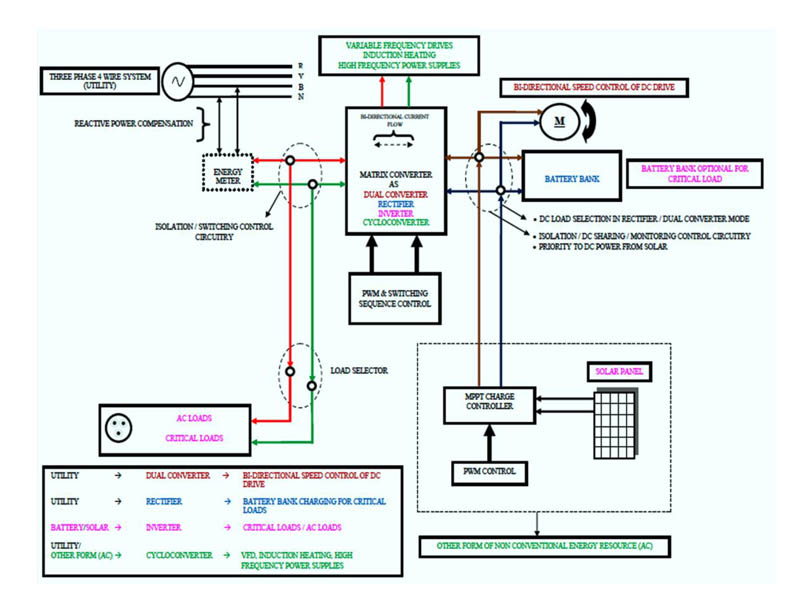Fig. 1. Birds’ Eye View of Matrix Converter Applications…

The unchanged system could also be implemented as Dual Converter only by changing the switching algorithm to drive DC motor in both the directions. This must be ensured with necessary isolation between the battery load and the motor load when implementing as a system on whole for every function (rectifier or dual converter).

Matrix Converter Topology

The Matrix Converter topology in a single phase consists of four bidirectional switches as matrix relating the supply input to the load output at the intersections as shown in Figure 2.Fig. 2. Matrix Converter Topology…

The bidirectional switches (Figure 3) are capable of conducting the current in both directions while at the same time blocking voltage. The Matrix Converter topology comprising the bidirectional switch is presented in Figure, in which S1 is mentioned as S1a & S1b, S2 as S2a & S2b, respectively. The input to the Matrix Converter may be AC or DC depending upon the make use of the Matrix Converter as particular power electronic converter.Fig. 3. Bidirectional Switch of Matrix Converter…

Functional Possibilities with Operational Sequence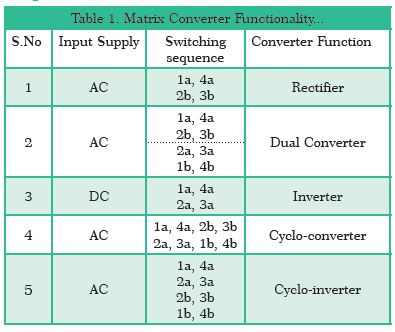Matrix Converter as Rectifier

The Matrix Converter is functional as Rectifier-Inverter system along with solar, with priority given to solar power conversion system for charging the battery as well as to
meet out the load and compensation via utility. Based on the requirements, both the applications could be incorporated in a single Matrix Converter system with proper load isolation between the load and Battery.

Let the supply input to the Matrix Converter as shown in Figure 2 is AC. For the positive half cycle of the AC input the switches 1a and 4a are triggered as given in Table 1. Then for the negative half cycle of the input, the other two pairs 2b and 3b are triggered such that the output from the Matrix Converter is DC. This is clearly presented by the simulation results of MATLAB/Simulink.Fig. 4. Simulation Circuit of Matrix Converter as Rectifier…Fig. 5. Input Voltage vs Output Voltage for Matrix Converter as Rectifier…

The Figure 4 shows the simulation circuit of Matrix Converter working as rectifier with input AC supply of 230Vrms and the output is DC of the same value (Figure 5). The analysis is carried out for resistive load. The gate pulses are developed by SPWM technique and are given to the switches 1a, 4a and 2b, 3b as per the sequence shown in Table 1.

Matrix Converter as Dual Converter

Here the supply input to the Matrix Converter as shown in Figure 1 is AC. The Matrix Converter is made to function as dual converter for bidirectional speed control of DC drives. For the positive half cycle of the AC input, the switches 1a and 4a are triggered. Then for the negative half cycle of the input, the other two switches of the pair 2b and 3b are triggered such that the output from the Matrix Converter is positive DC voltage. For the
same positive half cycle of the AC input, if the switches 2a, 3a are triggered and for the negative half cycle of the input, the switches 1b, 4b are triggered, then the output from the Matrix Converter is negative DC voltage. This is also clearly presented by the simulation results of MATLAB/Simulink.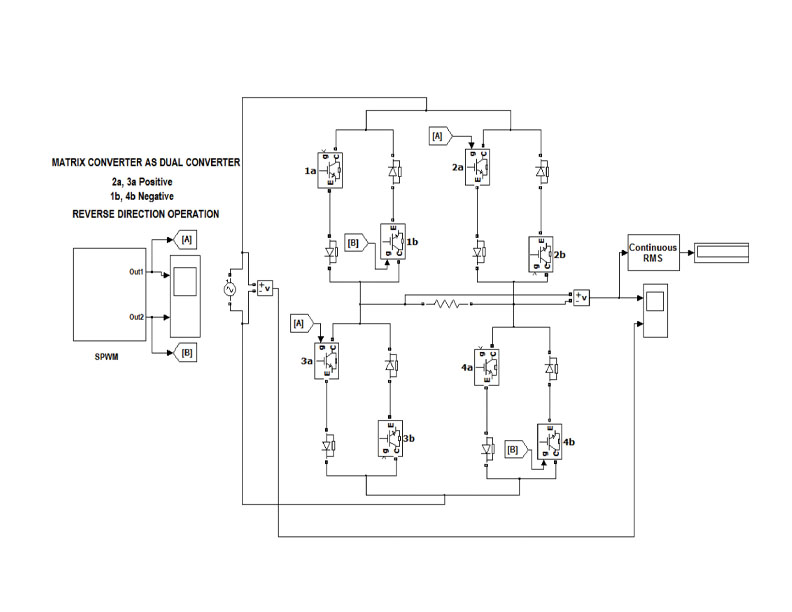Fig. 6. Simulation Circuit of Matrix Converter Operating as dual Converter…

The circuit of Matrix Converter in Matlab simulation is shown in Figure 6 for its operation as dual converter in which the switching sequences are noticeably given in Table 1. The positive and negative output DC voltage with respect to input is given in Figure 7 and 8.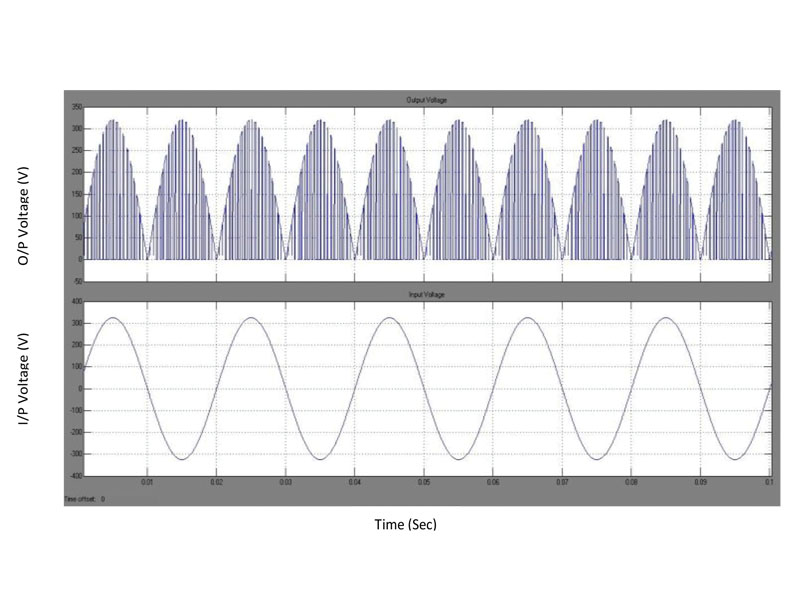Fig. 7. Positive DC Output Voltage vs Input Voltage…Fig. 8. Negative DC Output Voltage vs Input Voltage…Fig. 9. Simulation Circuit of Matrix Converter as Inverter…Fig. 10. Simulation Circuit of Matrix Converter as Cyclo-Converter…

Matrix Converter as Inverter

The Matrix Converter as inverter could possibly be made to drive AC motor with its variable frequency inverted AC output as speed control of AC drive via changing the frequency. For the circuit shown in Figure 2, if the input to the Matrix Converter is DC and the switching
sequence are given as 1a, 4a and 2a, 3a then the Matrix Converter will function as inverter giving AC output of the frequency as that of the PWM frequency. The simulation circuit as well as the result is shown below in Figure 9 and Figure 10.

The inverter operation of Matrix Converter could be applied to get the AC output voltage of the required frequency by varying the frequency of the PWM with that of the reference cycles. This operation could be implemented for speed control of AC drives via the frequency control of the output AC voltage. The input DC to the Matrix Converter may optionally be from the solar power conversion system, so that the battery is utilized only in case of emergency/ critical loads.

Matrix Converter as Cyclo-converter

The operation of the Matrix Converter as Cycloconverter for positive output load voltage is obtained by the conduction of the switches 1a, 4a for the positive half cycle of the AC input, and 2b & 3b for the negative half cycle of the input and similarly 2a, 3a, & 1b, 4b for
the negative output load voltage. For Cyclo-converter mode of operation, the output frequency obtained is given by, fo = fin/Nr, Where, fo = Output frequency, fin = Input frequency and Nr = Real number. The operation of Matrix Converter as Cyclo-converter could be easily understandable from the following simulation circuit and outputFig.11. Simulation Circuit of Matrix Converter as CycloConverter…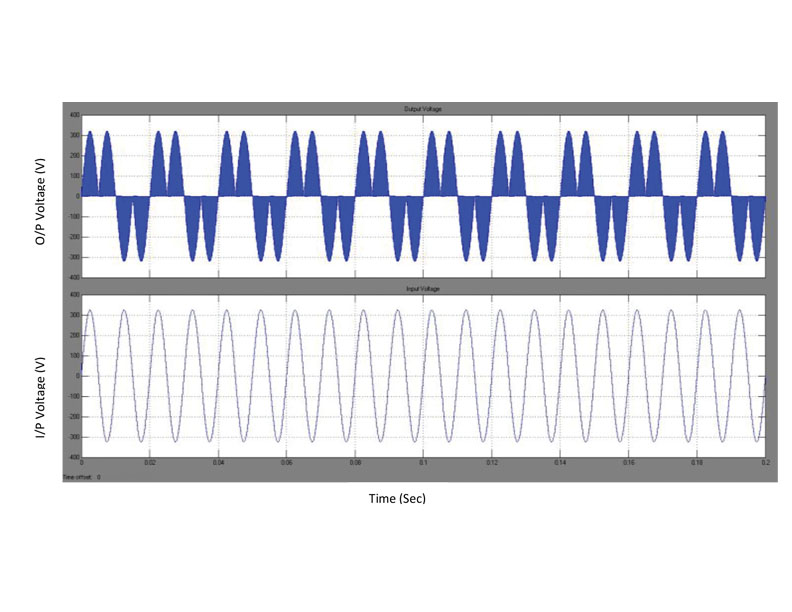Fig. 12. Simulation Output of Cyclo-Converter (100Hz Input Voltage vs 50Hz Output Voltage)…

Here, the output frequency is given by, fo = fin/ Nr, 50=100/2, in which the desired output is achieved by the SPWM pulses of the reference frequency of 50Hz. Figure.12 shows the AC output voltage of 50Hz waveform from the 100Hz AC input waveform.

Matrix Converter as Cyclo-inverter

Similarly the operation as Cyclo-inverter is obtained by the conduction of switches 1a, 4a, 2a, & 3a for the positive half cycle of the input and 2b, 3b, 1b and 4b for the negative half cycle of the input in which the output frequency fo will be fo = fin x Nr, Where, fo = Output
frequency, fin = Input frequency and Nr = Real number. The output frequency of the Matrix Converter when working as Cyclo-inverter will be of the necessary value depending on the developed SPWM cycle frequency.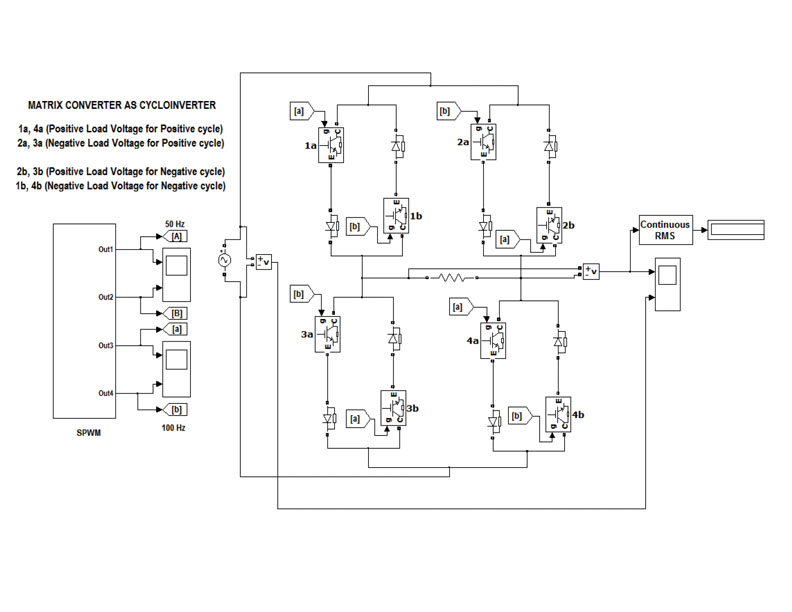Fig. 13. Simulation Circuit of Matrix Converter as Cyclo-Inverter…

Figure 13 shows the simulation circuit of the Matrix Converter and followed by is the simulation result (Figure 14) in which the output frequency is 100Hz for an input of 50Hz AC supply.

Thus, the Matrix Converter could be implemented as Cyclo-inverter for developing the output of desired frequency with that of the input via SPWM technique. For cyclo-converter and cyclo-inverter process the input AC source could necessarily be either from utility or
other non-conventional form of AC source like wind, etc. The possibility of combinational functionalities of Matrix Converter could enhance the power to weight ratio. The change of sequence from Rectifier to Inverter should start only if the utility power fails (offline) and a necessary monitoring / control circuit for that should be provided and vice versa for the change-over from Inverter to Rectifier operation. Generation of switching sequence can be implemented through SPWM as it is immune to noise and less susceptible to voltage changes. A Microcontroller or advanced controller like FPGA can play an effective role in generation of PWM pulses, changing over the sequence and for monitoring the controllability over it. Necessary protection (back from Matrix Converter to line) / isolation can also be implemented with proper isolation control circuitry employing digital controllers.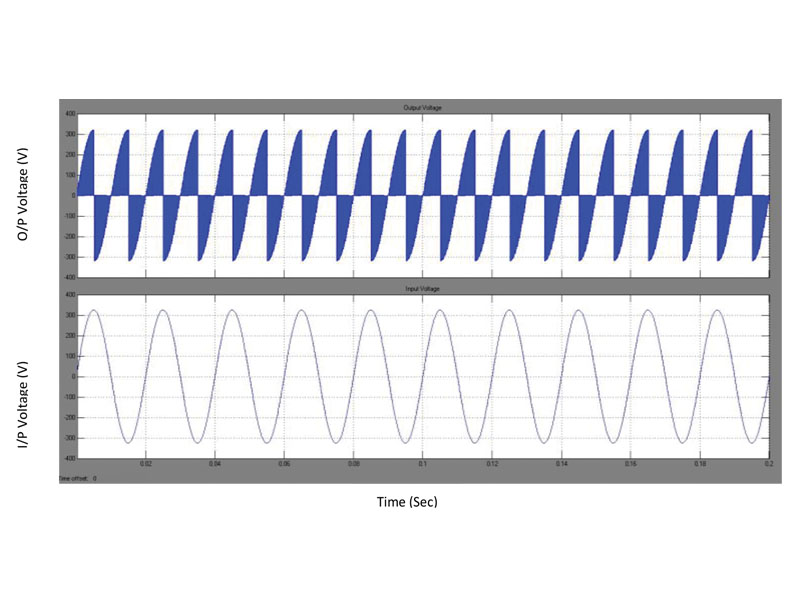Fig. 14. Simulation Output of Cyclo-inverter (100 Hz Output Frequency vs 50 Hz Input Supply)…

Matrix Converter as a Z-source Buck-Boost Converter

Minh-Khai Nguyen et al represent Single Phase Matrix Converter as a Converter that can buck and boost with step-changed frequency and both the frequency and voltage can be stepped up or stepped down. The Converter is employed with a safe-commutation strategy
to conduct along a continuous current path eliminating voltage spikes on the switches, thus avoiding snubber circuit. The authors describe the construction of the circuit with an LC input filter, a Z-source network, bidirectional switches and an RL load. The sequence
of switching control is in two states, where state 1 includes active mode and commutation mode and state 2 with shoot-through mode, free-wheeling mode and commutation mode. Here the output voltage can be produced at three different frequencies 120, 60 and 30
Hz in the buck-boost amplitude mode. This application of Single Phase Matrix Converter as a Z-source BuckBoost Converter is suitable for controlling the speed of a fan or a pump without the use of an inverter as in conventional AC-DC-AC systems.

Matrix Converter as a Four Quadrant DC Chopper

The Matrix Converter in single phase can also be implemented as a Four Quadrant DC Chopper. A four Quadrant DC Chopper (FQDC) converts a fixed DC into a variable DC output statically. Here, the magnitude of the output voltage of the converter is controlled by PWM and quadrant operation is due to the bidirectional control of current in switches of the Matrix Converter. A dead time is introduced in the switching transitions to allow the dissipation of energy in the load inductance. Mohammad Noor S. Z. et al have studied
the principles of four quadrant DC-DC Chopper and the switching sequence has been realized in the FPGA for the implementation of DC chopper using Single Phase Matrix Converter.

Matrix Converter as a Quasi-Z-source AC-AC Converter

Minh-Khai Nguyen et al. had represented single phase Matrix Converter as quasi-Z-source ac-ac converter. This converter consists of all the possibilities of traditional single-phase Z-source ac-ac converter like buck-boost, reversing, or maintaining the phase angle, and more specially sharing the same ground and operating in continuous current mode. The author had reported the converter with high efficiency, lower input current harmonic distortion and higher input power factor. Further as the converter employs duty-ratio control, it can also be implemented as DVR to compensate for voltage sags and swells in the ac-ac line conditioning. The authors represent the quasi-Z-source ac-ac converter as
a modified one with the following features as the output voltage bucked or boosted and be both in-phase and outof-phase with the input voltage sharing the same ground.

Matrix Converter as an Off-grid solar photovoltaic power system

T. R Sumithira et al represent the implementation of single phase Matrix Converter for an off-grid solar photovoltaic power system as it would be a feasible solution for medium power applications at remote locations without electricity connection in the state of Tamil Nadu. The authors claim a THD of less than 7% with the implantation of Matrix Converter. Here the matrix Converter in single phase is implemented as an inverter with Modified Pulse Width Modulation MPWM. FPGA is used here to realize the modulation strategy and
commutation technique for SPMC.

Matrix Converter for Wind Energy Conversion System

Navdeep Singh et al had explored an alternative to multistage AC-DC-AC power conversion. Matrix Converter is used as a single stage AC-AC power converter for interfacing the wind energy conversion system (WECS) to a grid as a distributed load system. In this, a control mechanism and time switching pattern are employed to convert variable frequency input power which is proportional to wind speed into a constant frequency output power for a distributed load system in a single unit. The authors justify that this system is suitable for interfacing a wind turbine to a distributed load system technically with adaptive active and reactive power injection control.

(To be continued in the next issue…)K. Vijayakumar possesses an M.E Degree from Anna University, Chennai. He is
presently working as an Assistant Professor and pursuing research in the area of Power
Electronics in the Department of EEE, Kalasalingam University, Krishnankoil – 626190, India,S. Kannan received PhD Degree from Madurai Kamaraj University. He is a Professor and Head of Electrical and Electronics Engineering, Ramco Institute of Technology, Rajapalayam – 626117, India.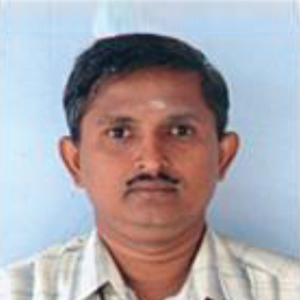S. Ponnayira Sundaravel is BE in Electrical and Electronics Engineering from Anna University, Chennai. He is presently working as Assistant Professor in the Department of EIE, Kalasalingam University, Tamil Nadu – 626190, India.